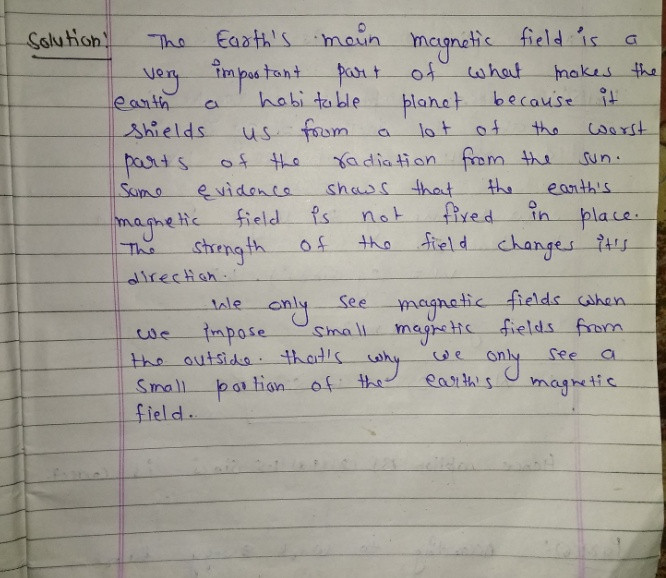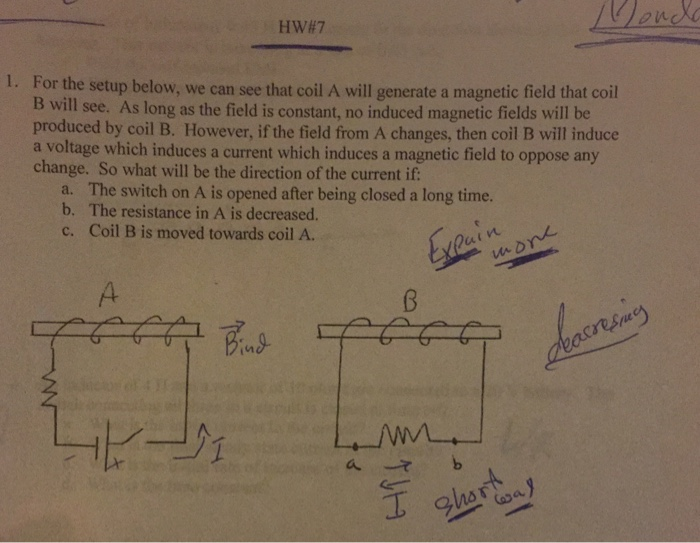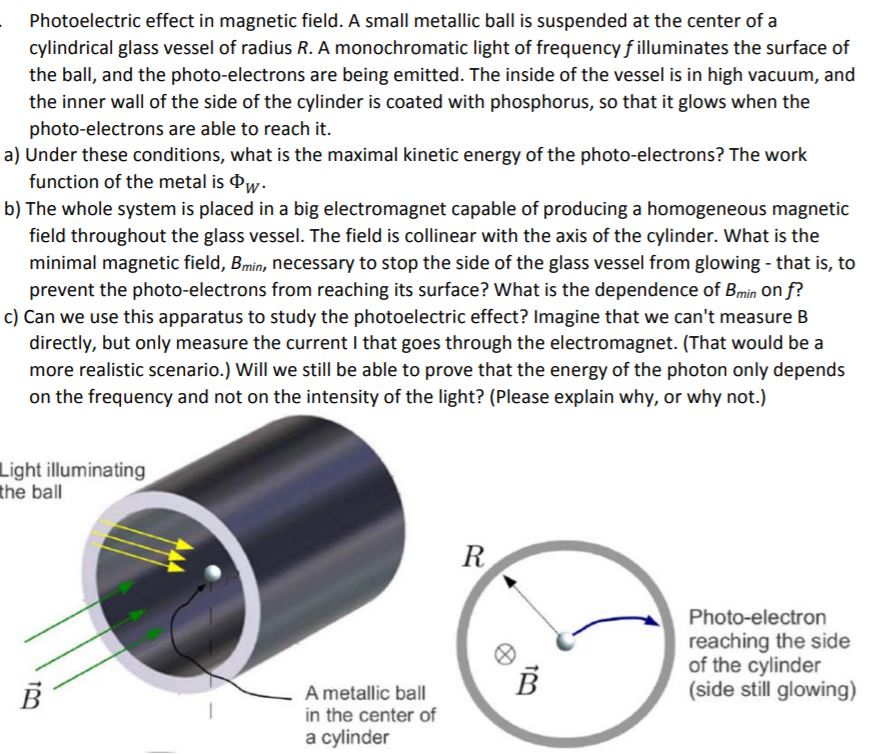Question

# Why do we only see a small portion of the earth’s magnetic field?

Why do we only see a small portion of the earth’s magnetic field?#### Earn Coins

Coins can be redeemed for fabulous gifts.

Similar Homework Help Questions
• ### help please 2. Why do we see that each cell is only expressing a small fraction...help please 2. Why do we see that each cell is only expressing a small fraction of the genes in it's genome at any given time?

• ### You want practically no magnetic field in a small region of space, not more than 10%...

You want practically no magnetic field in a small region of space, not more than 10% of Earth’s magnetic field at that location. The Earth’s magnetic field line is angled downward 72.7 degrees into the earth but practically due north. The field strength is 55.66 microTesla a. Take a single wire and make a loop with it with a radius of 80 cm. Put your small apparatus right at the origin of this loop. This will work because it is...

• ### At a location near the equator, the earth’s magnetic field is horizontal and points north. An...

At a location near the equator, the earth’s magnetic field is horizontal and points north. An electron is moving vertically upward from the ground. What is the direction of the magnetic force that acts on the electron? (a) North (b) East (c) South (d) West (e) The magnetic force is zero.

• ### For the setup below, we can see that coil A will generate a magnetic field that...For the setup below, we can see that coil A will generate a magnetic field that coil B will see. As long as the field is constant, no induced magnetic fields will be produced by coil B. However, if the field from A changes, then coil B will induce a voltage which induces a current which induces a magnetic field to oppose any change. So what will be the direction of the current if: The switch on A is opened...

• ### #2 near the ground at the north magnetic pole, the earth’s magnetic field is directed downward vertically and has a str...

#2 near the ground at the north magnetic pole, the earth’s magnetic field is directed downward vertically and has a strength of 0.57 G. An electron at the north pole is projected from the ground into this magnetic field with a velocity of 1.4 km/s vertically upward and 1.9 km/s horizontally to your right. A) find the magnitude of the force acting on this electron B) find the electron’s acceleration (magnitude and direction)

• ### the earth’s magnetic field is about 50 µT, at a 60° dip angle. If your textbook...

the earth’s magnetic field is about 50 µT, at a 60° dip angle. If your textbook is resting on the table, what is the downward flux through the cover of the book?

• ### A cosmic-ray electron moves at 7.0 × 106 m/s perpendicular to Earth’s magnetic field at an...

A cosmic-ray electron moves at 7.0 × 106 m/s perpendicular to Earth’s magnetic field at an altitude where the field strength is 0.5 × 10−5 T. What is the period of revolution of electron? in microseconds.

• ### Suppose you wanted to use Earth’s magnetic field to make an ac generator at a location...

Suppose you wanted to use Earth’s magnetic field to make an ac generator at a location where the magnitude of the field is 0.05000 mT. Your coil has 1008 turns and a radius of 3.700 cm. At what angular velocity would you have to rotate it in order to generate an emf of amplitude 1.000 V?

• ### Suppose you wanted to use Earth’s magnetic field to make an ac generator at a location...

Suppose you wanted to use Earth’s magnetic field to make an ac generator at a location where the magnitude of the field is 0.05000 mT. Your coil has 1062 turns and a radius of 3.600 cm. At what angular velocity would you have to rotate it in order to generate an emf of amplitude 1.400 V?

• ### Photoelectric effect in magnetic field. A small metallic ball is suspended at the center of a...Photoelectric effect in magnetic field. A small metallic ball is suspended at the center of a cylindrical glass vessel of radius R. A monochromatic light of frequency f illuminates the surface of the ball, and the photo-electrons are being emitted. The inside of the vessel is in high vacuum, and the inner wall of the side of the cylinder is coated with phosphorus, so that it glows when the photo-electrons are able to reach it. a) Under these conditions, what...